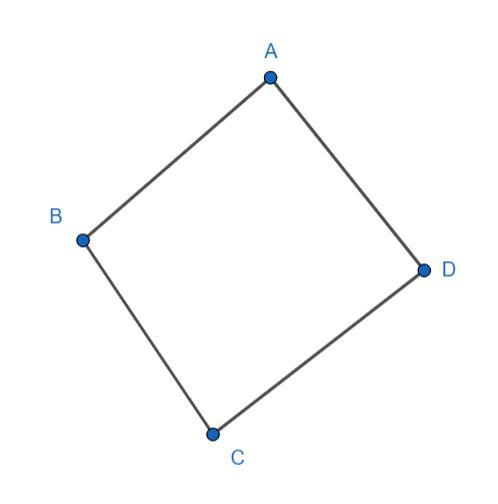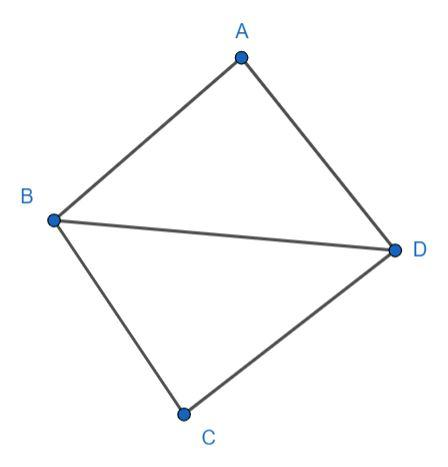Courses
Courses for Kids
Free study material
Offline Centres
MoreLast updated date: 03rd Dec 2023
Total views: 381.6k
Views today: 4.81k

# What is the sum of the measures of the angles of a convex quadrilateral? Will this property hold if the quadrilateral is not convex?Verified
381.6k+ views
Hint: Recall what is a quadrilateral and its properties. Then, find the sum of the measures of the angles of the convex quadrilateral. Check if the property holds if the quadrilateral is not convex.

We know that a quadrilateral is a closed polygon with four sides. A convex quadrilateral is defined as having its angles, each, less than 180° and all its diagonals lying inside it.

Consider a convex quadrilateral ABCD as shown in the figure.We have to find the sum of the measures of the angles of this quadrilateral ABCD.

We can join points B and D, to construct a diagonal BD, which divides the quadrilateral ABCD into two triangles.We know that the sum of measures of the angles of a triangle is 180°. We have two triangles ABD and CBD, therefore, the sum of the measures of the angles of the convex quadrilateral is two times the sum of the measures of the angles of the triangle which is 360°.

Therefore, the sum of the measures of the angles of the convex quadrilateral is 360°.

We can divide any quadrilateral into two triangles. Hence, the sum of the measures of the angles of any quadrilateral is 360°.
Hence, the property holds for any quadrilateral.

Note: You can choose any convex quadrilateral, it is not necessary for it to be a regular quadrilateral. You can divide the quadrilateral into two triangles into two ways by constructing one diagonal out of the two.